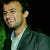# Embeddings & Word2Vec

Source: Deep Learning on Medium

Word-embedding is the collective term for models that map a set of words or phrases to vectors of numerical values. These vectors are called embedding and in general the word-embedding are used to reduce the dimensionality of the text data. These embeddings can also learn the traits of the given words. The embeddings can learn the traits that are present in the training data.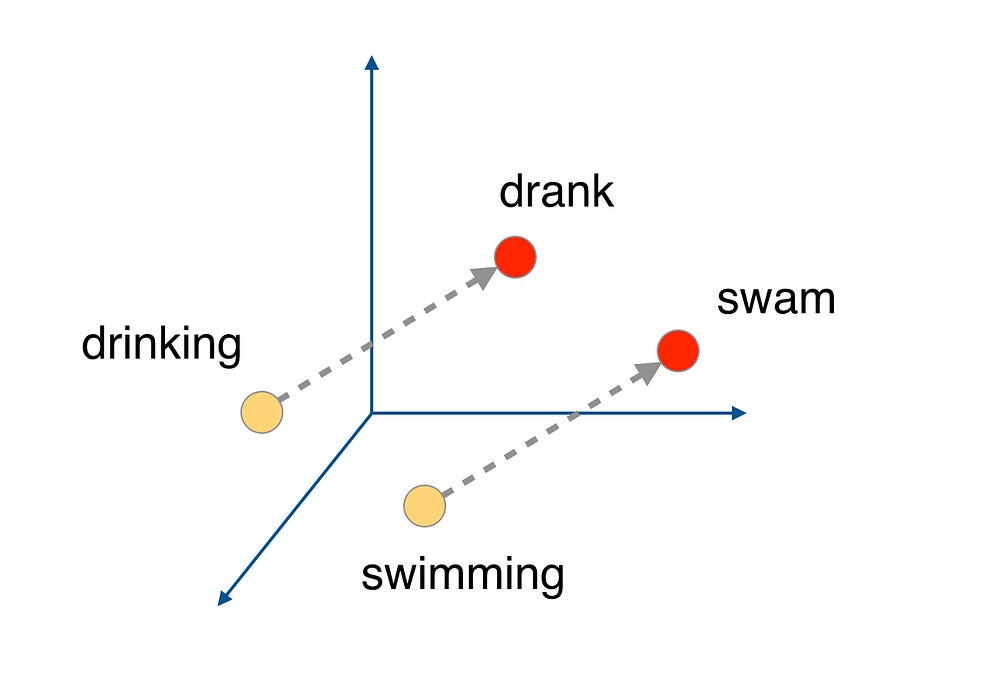Figure 1. Relation captured by Word-vectors

When we change the test data to words, we have thousands of words, and one-hot encoding them results in a vector with mostly zeros, and only one 1 in that vector. The output is calculated by multiplying the input one-hot vector with the weight matrix, the product of the matrix thus obtained is large with mostly zeros.
With the embedding layer we can perform the matrix multiplication efficiently by grabbing the row of the embedding-weight matrix that corresponds to the index of the value whose value is 1.
So we can represent the weight matrix as a look-up table and we can map the words to integers.
Embedding layer is just a hidden layer and the embedding matrix is just a weight matrix.
Word2Vec says that any word that appear in similar context in a text should have similar representation. Context here means the word that appears before or after the word in consideration.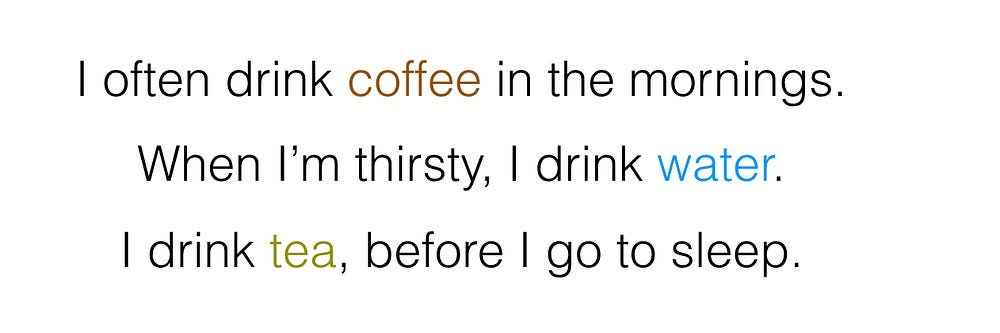Figure 2. Coffee, water and tea are said to appear in similar context

So, while training the word2vec, we provide the context(the surrounding words) as input and then try to predict the missing word. This approach is called continuous bag of words or the CBOW model.

Another approach is to input the word of interest and predict the context words. This is called the skip-gram model.

After looking at both of these models we try and formalize the idea of context by defining the term window-size.

To follow along the implementation of word2vec, as described in this notebook, we have to run this notebook locally, or use this kaggle-kernel, with GPU for development and training.

There are certain words that will show-up anywhere, so we should make sure not use them in the context, as they will add noise to our presentation. So, if we can discard some of these words(very frequently-occurring words) then it will speed up training and reduce the involvement of noise. This process of discarding words is known as Sub-sampling. If we discard the word “the” using the sub-sampling, then the probability of discarding the word is given fig. 3.Figure 3. 0(zero) is the index of “the” 16*10⁶ is total number of words and 1*10⁶ is the total occurrence of “the”

After discarding there will be 12,000 of the original 1*10⁶ “the” in text. The idea behind sub-sampling is to get rid of most of the frequently occurring words while retaining enough words to learn the embedding.

We use the following code snippet to generate the context of a given word.

Now to generate the batch of words and their corresponding context-words, we use the following code-snippet.

Below we show the graph that represents the architecture of the network, we use to train our model.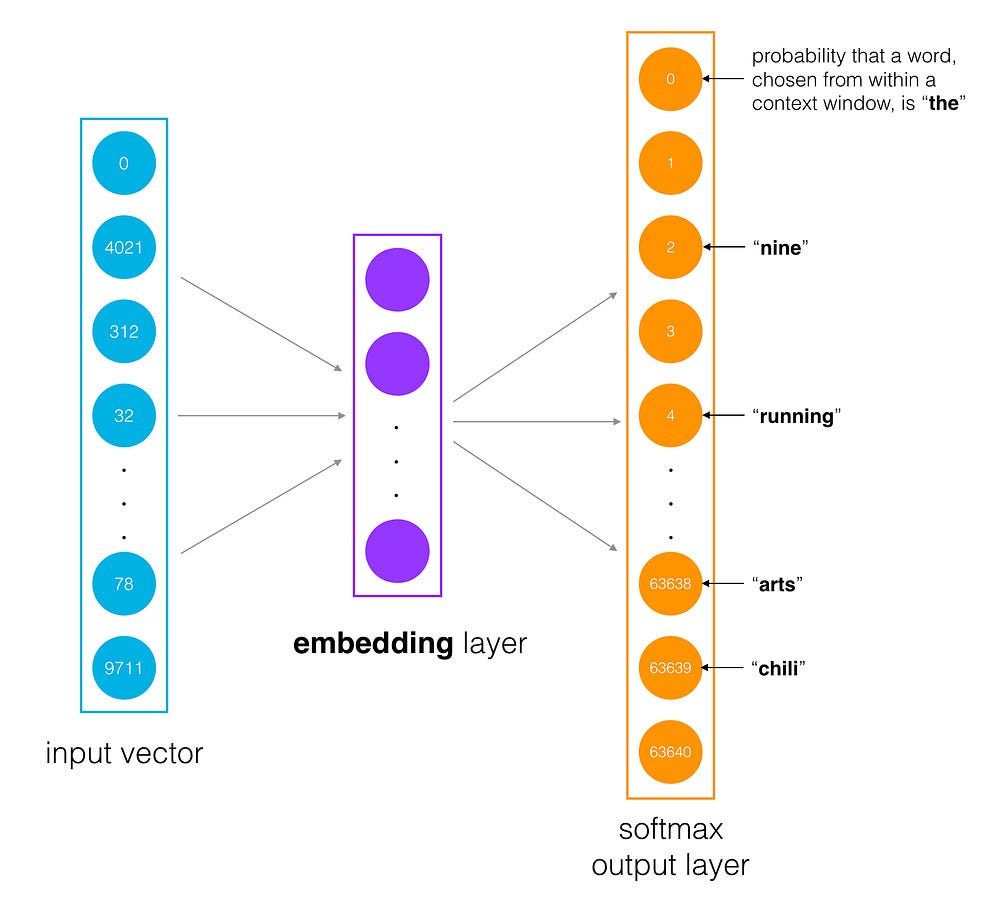Figure 4. Network architectures, used to train the word-embedding

Once we train the model we can get rid of the last layer and keep the embedding to perform some interesting mathematical operations in vector space, that will show relation between the word-vectors.

While defining an embedding layer we take help of the PyTorch embedding layer, which is a sparse layer type. The two parameters that it accepts are, number of rows in the embedding (corresponding to the the number of words under consideration) and number of columns in the embedding (corresponding to the dimension of embedding-vector).

The following code snippet shows the model architecture, which is used for training the word-embedding.

After training the word-embedding we plot them using T-SNE in two dimensions. Following image shows the 2-dimensional plot.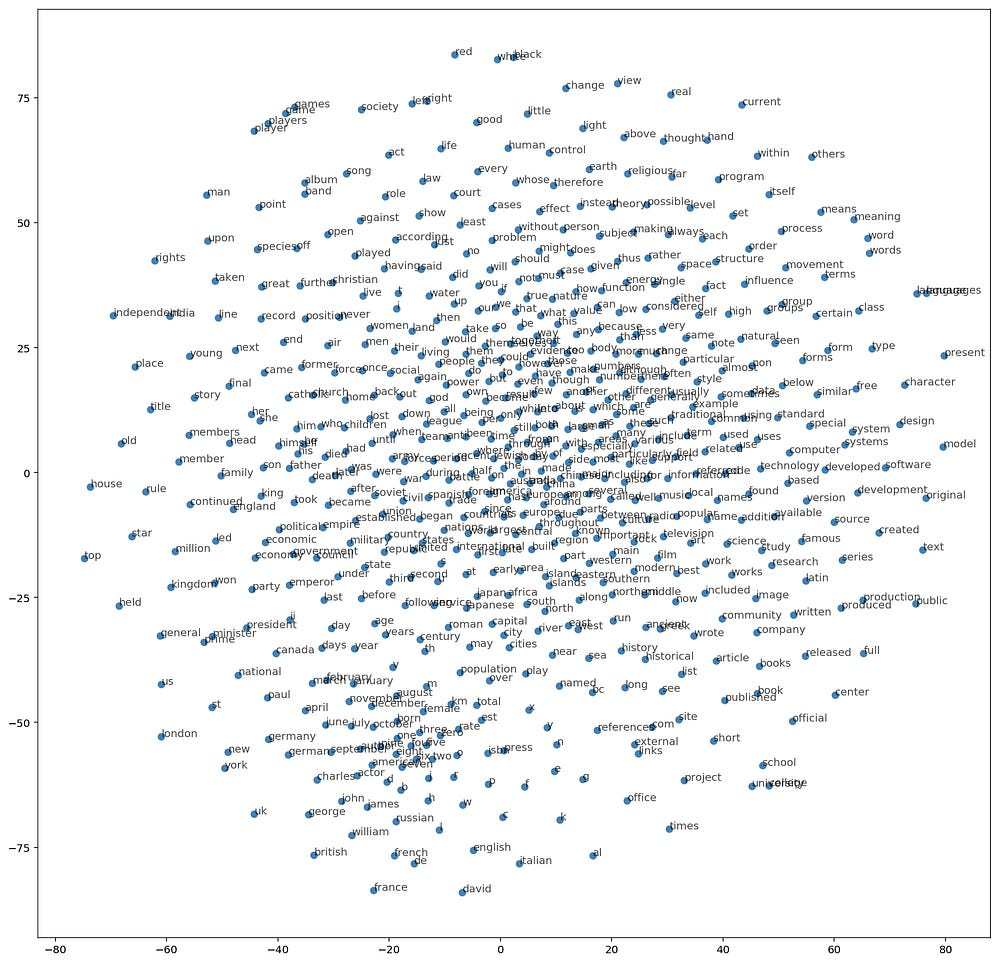Figure 5. Plot showing 2-dimensional representation of the word-vectors

This story was developed as a part of self-learning exercise for the Deep-Learning nanodegree, for which scholarship was provided by Udacity and Facebook. I thank https://twitter.com/cezannecam for explaining the underlying concepts. Contents of the above stories are borrowed from the contents provided by Udacity for the stated course.